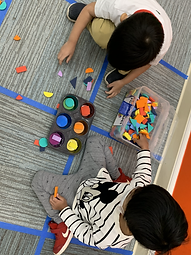Ms. Kayla

Target 1​

Lesson Type:

Continuation

Number Operation

:

Number System

Understand that numbers have an order (limited to numbers 0 to 50).

1:

Understand that the number sequence does not change, even when some numbers are missing from a given sequence.

2:

Understand that the last number counted depicts the quantity of the objects.

3:

Identify when a given sequence is a correct count sequence.

Pre-K

Vocabulary:

Count, Number

Activities:

1. Students were given an image with a given number of creatures. Students had to count and represent the number numerically.

2. Students also made little number booklets to quantify numbers on each page.Home Exploration

Guiding Questions:Absent Students:

Target 2

:

Pre-K

Vocabulary:

Pattern, Color

Activities:Home Exploration

Guiding Questions:Target 3

:

1:

Independently identify squares, rectangles, circles, and triangles.

2:

Identify basic geometric shapes that best represent real-world objects.

Pre-K

Vocabulary:

Shape, Triangle, Square, Rectangle, Circle

Activities:

1. Students create real-world objects using the foam shapes (example: tree, house, car, sun).

2. Students also sorted the shapes by one attribute - color.Home Exploration